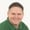Lessons: 11Length: 46 minutes• Overview
• Transcript

# 3.3 Class Constant Visibility

As of PHP 7.1, we can now modify the accessibility of class constants. It's about time...

## 4.Conclusion1 lesson, 01:09

### 3.3 Class Constant Visibility

Classes in PHP have allowed us to modify the accessibility of properties and methods, and for example let's write a very brief class called calculator and one of the things a calculator needs to give us access to is the ability to add two numbers together. So you would have public function and let's just work with its, and so he would have int A int B and then of course we would simply return a plus B. So there's nothing new there. However, when it came to constants instead of a class. Well there was no way that we could define the accessibility of a constant if we created a constant it was publicly accessible. So I'm going to call this PUBLIC_CONSTANT and it's going to be a value of 1. So with PHP 7.1, this has changed. We can now modify the accessibility of a constant inside of a class. So if we wanted to be explicit and say that this is a public constant, we could do that. But we can also declare a private constant and let's just call that private constant and we'll set that equal to two. We also have the ability to set a protected constant. So just like our properties and methods, we can have public private and protected constants and let's go ahead and give that a value of 3. So lets var_dump this will save var_dump and we want to use calculator and let's start with public constants, now we of course are going to see the value of one inside of the console. Now Visual Studio code does not like this syntax because the PHP plugin does not yet support 7.1, but if we run this we see the value of 1. So yes that of course worked. However if we try to access the private constants, well, we're going to run into an error. We cannot access the private constant of private constant. And we're going to see the same thing for protected as well. So, once again uncut error although this says undefined class constant. So that is definitely not correct and well why isn't that? That's why. So if we run this again then we cannot access the protected const of protected constant. So, out of all of the new features, this is obviously the easiest. Constants inside of our classes are now just like our properties and methods. We can define them as public, private, and protected.

Back to the top